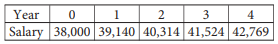mycollegehive
The table above shows the yearly salary, in dollars, of an ...
PSAT PSAT-math-test math0The table above shows the yearly salary, in dollars, of an employee at a company. Which of the following best describes the type of model that fits the data in the table?

A) Linear, increasing by approximately \$1,140 per year

B) Linear, increasing by approximately \$1,245 per year

C) Exponential, increasing by approximately 3% each year

D) Exponential, increasing by approximately 9% each year

130 viewsShareFollowSchool not set Nigeria
06 January 2021University of Benin Nigeria
06 January 20210First, calculate the yearly increase in salary.

If the increase is constant through out each year, a linear model can be applied.

From Year 0 to Year 1, the salary increases by:

39,140 - 38,000 = \$1,140

From Year 1 to Year 2, the salary increases by:

40,314 - 39,140 = \$1,174

From Year 2 to Year 3, the salary increases by:

41,524 - 40,314 = \$1,210

From Year 3 to Year 4, the salary increases by:

42,769 - 41,524 = \$1,245

Since the rate of increase is not constant, a linear model cannot be applied, thus, an exponential model is used instead.

Next, calculate the increase ratio using any year range.

Using year 0 to year 1,

Increase ratio = 39,140/38,000

= 1.03 OR 3%Share

### Groups

How to insert math formulas/equations### Related Tags

PSAT

3 followers

94 questionsPSAT-math-test

4 followers

94 questionsmath

23 followers

1262 questions